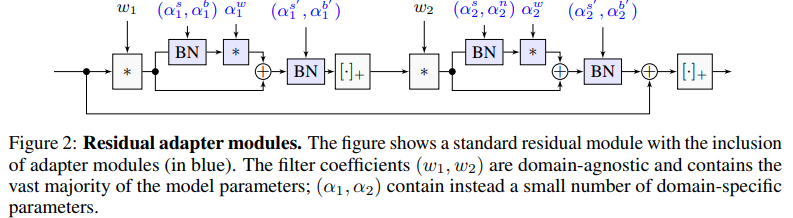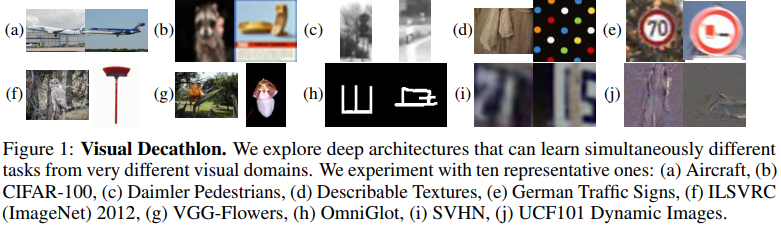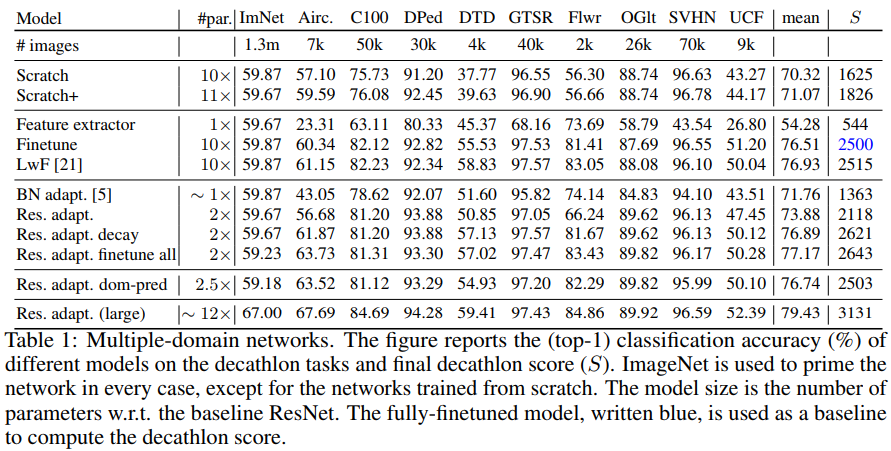## Summary

The goal of this paper is to develop neural network architectures that can work well in a multiple-domain setting. The authors introduce a design for multivalent neural network architectures for multiple-domain learning (c.f. fig. 2). The key idea is to reconfigure a deep neural network to work on different domains as needed. They show that the proposed models are equivalent to packing the adaptation parameters in convolutional layers added to the network. The layers in the resulting parametric network are either domain-agnostic, hence shared between domains, or domain-specific, hence parametric.## Proposed method

As discussed in the paper, in one extreme, one could use 1 specific model per domain. In another extreme, we could use 1 model for every domain and just fine tune the last (classification) layer. The proposed solution lies between those two extremes. They start from a ResNet whose parameters are shared across every domain, i.e. they are domain-agnostic weights. To that resNet, they add Residual adapter modules which contain domain-specific weights (blue blocks in fig. 2). These modules are in the form

$g(x;\alpha) = x + \alpha * x$

where $$\alpha$$ is a $$1\times 1$$ filter.

They also add batch normalization modules after convolutional layers in order to normalize their outputs and facilitate learning (fig. 2). The normalization operation is followed by rescaling and shift operations $$s \odot x + b$$, where (s, b) are learnable parameters. In their architecture, they incorporate the BN layers into the adapter modules (fig. 2).

## ResultsThey tested their method on the Visual Decathlon challenge which combines ten well-known datasets from multiple visual domains. The show that their method is smaller and as good (if no better) than using 1 model per domain. It is also far better than a naive one model + fine tuning approach.